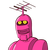# 1. Show that :(i) division of whole numbers is not closed.(ii) any whole number divided by 1, always gives the number

1. Show that :
(i) division of whole numbers is not closed.
(ii) any whole number divided by 1, always gives the number itself.
(iii) every non-zero whole number divided by itself gives 1 (one).
(iv) zero divided by any non-zero number is zero only.
(v) a whole number divided by O is not defined.
For each part, given above, give two suitable examples.
2. If x is a whole number such that x = x= x, state the value of x.​

### 1 thought on “1. Show that :<br />(i) division of whole numbers is not closed.<br />(ii) any whole number divided by 1, always gives the number”

1.